# PyTorch学习笔记(10)——上采样和PixelShuffle

》的论文。PyTorch 0.4.1将这些上采样的方式定义为Vision Layers，现在对这4种在PyTorch中的上采样方法进行介绍。

### 0. 什么是上采样？

Unpooling是在CNN中常用的来表示max pooling的逆操作。这是从2013年纽约大学Matthew D. Zeiler和Rob Fergus发表的《Visualizing and Understanding Convolutional Networks》中引用的：因为max pooling不可逆，因此使用近似的方式来反转得到max pooling操作之前的原始情况：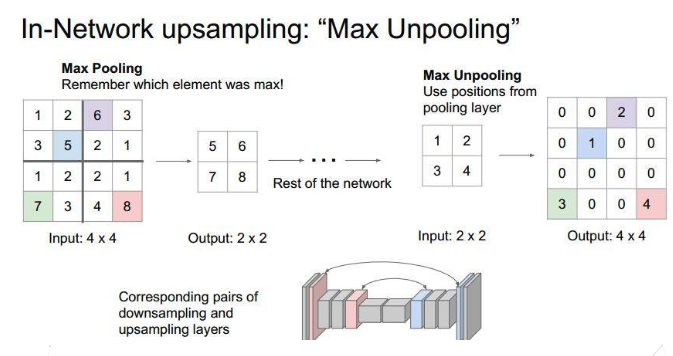Deconvolution(反卷积)在CNN中常用于表示一种反向卷积 ，但它并不是一个完全符合数学规定的反卷积操作。

Unpooling不同，使用反卷积来对图像进行上采样是可以习得的。通常用来对卷积层的结果进行上采样，使其回到原始图片的分辨率。

### 1. Vision Layer

• ① PixelShuffle
• ② Upsample
• ③ UpsamplingNearest2d
• ④ UpsamplingBilinear2d

#### 1.1 PixelShuffle

》的论文。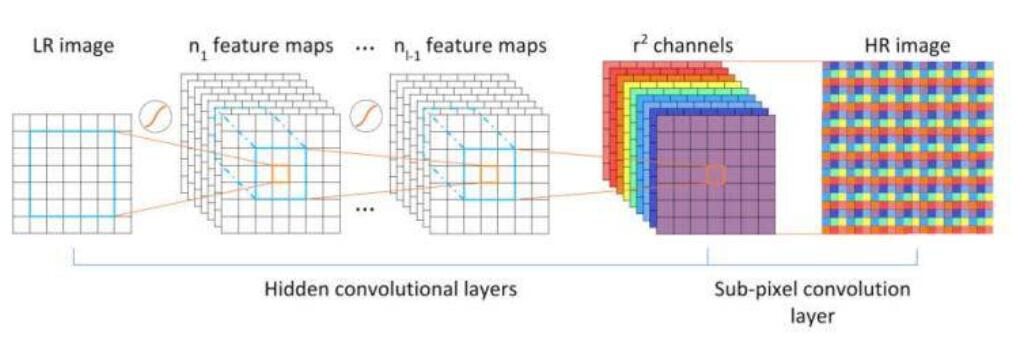pixelshuffle算法的实现流程如上图，其实现的功能是：将一个H × W的低分辨率输入图像（Low Resolution），通过Sub-pixel操作将其变为rH x rW的高分辨率图像（High Resolution）。

#### 定义

class torch.nn.PixleShuffle(upscale_factor)


• 输入: (N,C x upscale_factor$^2$,H,W)
• 输出: (N,C,H x upscale_factor,W x upscale_factor)

#### 例子

>>> ps = nn.PixelShuffle(3)
>>> input = torch.tensor(1, 9, 4, 4)
>>> output = ps(input)
>>> print(output.size())
torch.Size([1, 1, 12, 12])


3）反卷积以及pixelshuffle这几个知识点。

#### 定义

class torch.nn.Upsample(size=None, scale_factor=None, mode='nearest', align_corners=None)


• size 是要输出的尺寸，数据类型为tuple： ([optional D_out], [optional H_out], W_out)
• scale_factor 在高度、宽度和深度上面的放大倍数。数据类型既可以是int——表明高度、宽度、深度都扩大同一倍数；亦或是tuple——指定高度、宽度、深度的扩大倍数。
• mode 上采样的方法，包括最近邻（nearest），线性插值（linear），双线性插值（bilinear），三次线性插值（trilinear），默认是最近邻（nearest）。
• align_corners 如果设为True，输入图像和输出图像角点的像素将会被对齐（aligned），这只在mode = linear, bilinear, or trilinear才有效，默认为False。

#### 例子

>>> input = torch.arange(1, 5).view(1, 1, 2, 2).float()
>>> input
tensor([[[[ 1.,  2.],
[ 3.,  4.]]]])

>>> m = nn.Upsample(scale_factor=2, mode='nearest')
>>> m(input)
tensor([[[[ 1.,  1.,  2.,  2.],
[ 1.,  1.,  2.,  2.],
[ 3.,  3.,  4.,  4.],
[ 3.,  3.,  4.,  4.]]]])

>>> m = nn.Upsample(scale_factor=2, mode='bilinear')  # align_corners=False
>>> m(input)
tensor([[[[ 1.0000,  1.2500,  1.7500,  2.0000],
[ 1.5000,  1.7500,  2.2500,  2.5000],
[ 2.5000,  2.7500,  3.2500,  3.5000],
[ 3.0000,  3.2500,  3.7500,  4.0000]]]])

>>> m = nn.Upsample(scale_factor=2, mode='bilinear', align_corners=True)
>>> m(input)
tensor([[[[ 1.0000,  1.3333,  1.6667,  2.0000],
[ 1.6667,  2.0000,  2.3333,  2.6667],
[ 2.3333,  2.6667,  3.0000,  3.3333],
[ 3.0000,  3.3333,  3.6667,  4.0000]]]])

>>> # Try scaling the same data in a larger tensor
>>>
>>> input_3x3 = torch.zeros(3, 3).view(1, 1, 3, 3)
>>> input_3x3[:, :, :2, :2].copy_(input)
tensor([[[[ 1.,  2.],
[ 3.,  4.]]]])
>>> input_3x3
tensor([[[[ 1.,  2.,  0.],
[ 3.,  4.,  0.],
[ 0.,  0.,  0.]]]])

>>> m = nn.Upsample(scale_factor=2, mode='bilinear')  # align_corners=False
>>> # Notice that values in top left corner are the same with the small input (except at boundary)
>>> m(input_3x3)
tensor([[[[ 1.0000,  1.2500,  1.7500,  1.5000,  0.5000,  0.0000],
[ 1.5000,  1.7500,  2.2500,  1.8750,  0.6250,  0.0000],
[ 2.5000,  2.7500,  3.2500,  2.6250,  0.8750,  0.0000],
[ 2.2500,  2.4375,  2.8125,  2.2500,  0.7500,  0.0000],
[ 0.7500,  0.8125,  0.9375,  0.7500,  0.2500,  0.0000],
[ 0.0000,  0.0000,  0.0000,  0.0000,  0.0000,  0.0000]]]])

>>> m = nn.Upsample(scale_factor=2, mode='bilinear', align_corners=True)
>>> # Notice that values in top left corner are now changed
>>> m(input_3x3)
tensor([[[[ 1.0000,  1.4000,  1.8000,  1.6000,  0.8000,  0.0000],
[ 1.8000,  2.2000,  2.6000,  2.2400,  1.1200,  0.0000],
[ 2.6000,  3.0000,  3.4000,  2.8800,  1.4400,  0.0000],
[ 2.4000,  2.7200,  3.0400,  2.5600,  1.2800,  0.0000],
[ 1.2000,  1.3600,  1.5200,  1.2800,  0.6400,  0.0000],
[ 0.0000,  0.0000,  0.0000,  0.0000,  0.0000,  0.0000]]]])


#### 定义

 class torch.nn.UpsamplingNearest2d(size=None, scale_factor=None)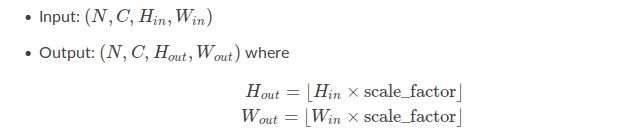#### 例子

>>> input = torch.arange(1, 5).view(1, 1, 2, 2)
>>> input
tensor([[[[ 1.,  2.],
[ 3.,  4.]]]])

>>> m = nn.UpsamplingNearest2d(scale_factor=2)
>>> m(input)
tensor([[[[ 1.,  1.,  2.,  2.],
[ 1.,  1.,  2.,  2.],
[ 3.,  3.,  4.,  4.],
[ 3.,  3.,  4.,  4.]]]])


#### 定义

 class torch.nn.UpsamplingBilinear2d(size=None, scale_factor=None)#### 例子

>>> input = torch.arange(1, 5).view(1, 1, 2, 2)
>>> input
tensor([[[[ 1.,  2.],
[ 3.,  4.]]]])

>>> m = nn.UpsamplingBilinear2d(scale_factor=2)
>>> m(input)
tensor([[[[ 1.0000,  1.3333,  1.6667,  2.0000],
[ 1.6667,  2.0000,  2.3333,  2.6667],
[ 2.3333,  2.6667,  3.0000,  3.3333],
[ 3.0000,  3.3333,  3.6667,  4.0000]]]])


### 2. 知识回顾

#### 2.1 Transposed convolution(转置卷积)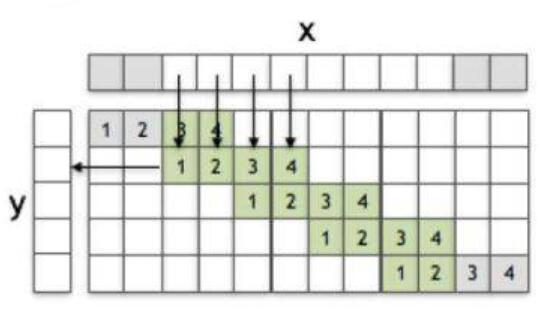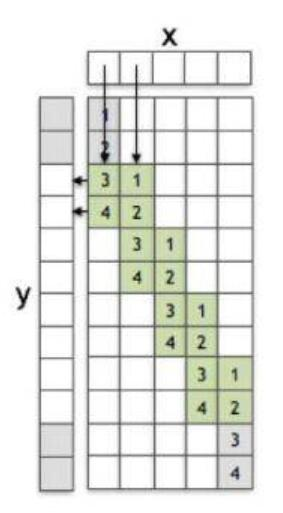$y_3$ = $3x_1 + x_2$

#### 2.2 Sub-pixel convolution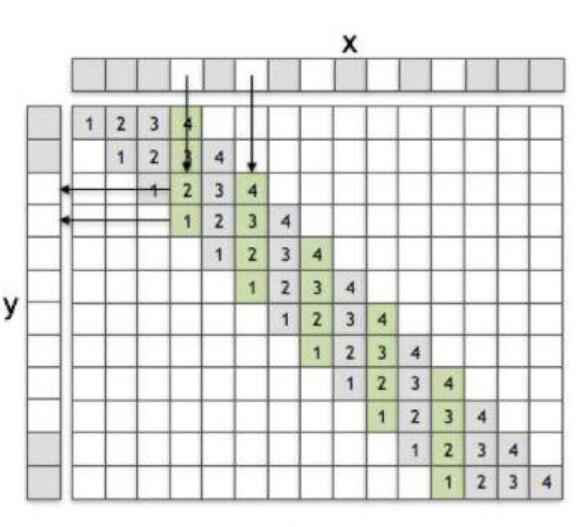1.1 PixelShuffle中说过，sub-pixel convolution的步长是介于0到1之间的，但是这个操作是如何实现的呢？简而言之，分为两步：

• ① 将stride设为1
• ② 将输入数据dilation(以stride=1/2为例，sub-pixel是将输入x的元素之间插入一些元素0，并在前后补上一些元素0)，或者说根据分数索引（fractional indices）重新创建数据的排列形式。

#### 2.3 Deconvolution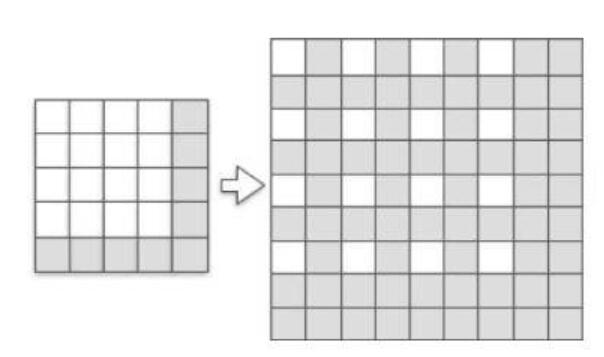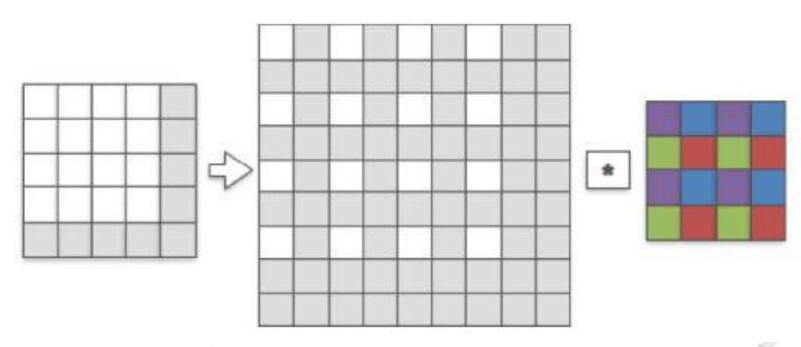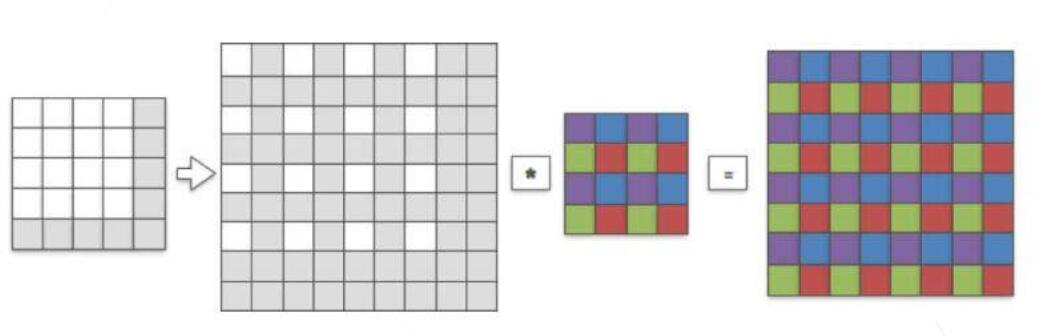Deconvlution的动态过程可见我之前翻译过的一篇文章《CNN概念之上采样，反卷积，Unpooling概念解释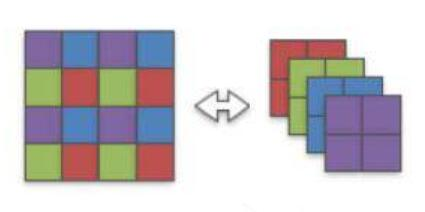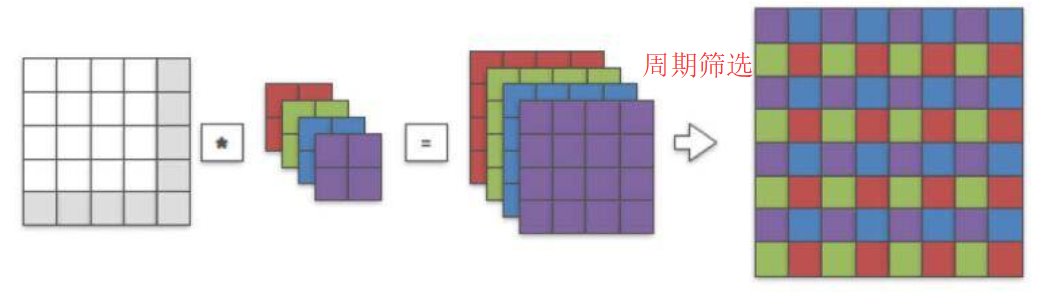### 4. 参考资料